Home > GHCN, trb > trb-0.07: Performance Refactoring

## trb-0.07: Performance Refactoring

2010 July 18

Introduction

I was hamstrung by slow performance. Too many ‘for loops’, not enough array processing. It wasn’t a problem until I tried a 36×72 grid with 2500 grid cells. Version 0.05 took 25 hours. I improved that yesterday in v 0.06: only 8 hours. But that still stank. This morning I finally found the right tool.

Method

I knew that ‘for loops’ are not the best answer in R-code. But I’m still way-down in that learning curve. I was looking at ways to use ‘factors’ to improve the processing when I finally realized that the answer was even simpler: tapply. And that reduces processing time to about 22 seconds (if you have previously written out the data files).

25 hours -> 22 seconds

Experienced R-coders can now punch me in the arm and say “Duh!”

```###############################################################################
# trb 0.07 : 2010 07 18 : Performance Refactor (22 seconds with data read)
# trb 0.06 : 2010 07 17 : Performance Refactor (3x faster, still 8hrs for all)
# trb 0.05 : 2010 07 14 : Gridding by Latitude and Longitude
# trb 0.04 : 2010 07 13 : Proportional Latitudinal Bands
# trb 0.03 : 2010 07 12 : Weighted Latitudinal Bands
# trb 0.02 : 2010 07 11 : Simple Latitudinal Bands
# trb 0.01 : 2010 07 10 : GHCN Simple Mean Average
# https://rhinohide.wordpress.com
###############################################################################
ver <- "0.07"
read <- FALSE # set to FALSE if this is your first time through
read <- TRUE # set to TRUE if you have previous data to read

###############################################################################
# Read Data Files
###############################################################################
# ftp://ftp.ncdc.noaa.gov/pub/data/ghcn/v2/v2.mean
# ftp://ftp.ncdc.noaa.gov/pub/data/ghcn/v2/v2.temperature.inv
###############################################################################

# this flag is to indicate if we must read for the first time
# or if we can read the data from previous runs

#------------------------------------------------------------------------------
# Temperature File (GHCN formatted monthly means)
#------------------------------------------------------------------------------

# mm for 'monthly means'
mmwids=c(11,1,4,5,5,5,5,5,5,5,5,5,5,5,5)
mmcols=c("id","dupe","year","jan","feb","mar","apr","may","jun",
"jul","aug","sep","oct","nov","dec")

# read the file
mm <- read.fwf("../data/v2.mean", widths=mmwids, col.names=mmcols,

#------------------------------------------------------------------------------
# Inventory File
#------------------------------------------------------------------------------

# there are five occurrances of the pound hash in v2.temperature.inv
# R scripts chokes on reading those, remove them first

invcols <- c("id","name","lat","lon","alt","elev","ru","pop","terr","arid",
invwids <- c(11,32,6,8,5,5,1,5,2,2,2,2,1,2,16,1,5)

###############################################################################
# Set up arrays for latitudinal banding
###############################################################################

hindex=new.env(hash=TRUE)
hlat=new.env(hash=TRUE)
hlon=new.env(hash=TRUE)
for (i in 1:nrow(inv)) {
hindex[[as.character(inv[i,1])]] <- i
hlat[[as.character(inv[i,1])]] <- inv[i,3]
hlon[[as.character(inv[i,1])]] <- inv[i,4]
}

mmlat <- rep(NA,nrow(mm))
mmlon <- rep(NA,nrow(mm))

for (i in 1:nrow(mm)) {
mmlat[i] <- hlat[[as.character(mm[i,1])]]
mmlon[i] <- hlon[[as.character(mm[i,1])]]
}

# add inv lat lon
mm1 <- cbind(mm,mmlat,mmlon)

# general formula for a set number of latitude bands of equal area
nbands <- 36
bands <- rep(NA,nbands)
b = 90                      # start with santa
bands=b
bands[nbands+1]=-90         # rounding errors at nbands ~ 32
for (i in 1:(nbands-1)) {
a = asin(sin(b*pi/180)-2/nbands)
b = a * 180 / pi
bands[i+1] = b
}
bands

# 'bars' are the longitudinal edges of the grid
# calculate the bars long now so we can just lookup later

nbars <- nbands * 2        # this could be set to any number
bars <- matrix(NA,nbands+1,nbars+1)
bars[,1] = -180            # start in Howland Island and move east ;-)
for (i in 1:(nbands+1)) {
for (j in 1:nbars) {
bars[i,j+1] = 360*(j/nbars)-180
}
}
bars[1,]
bars[nbands+1,]

#------------------------------------------------------------------------------
# assign each record to a grid point
#------------------------------------------------------------------------------
mmlat <- rep(NA,nrow(mm1))
mmlon <- rep(NA,nrow(mm1))
for (i in 1:nrow(mm1)) {
mmlat[i] = sum(bands>mm1[i,16])
mmlon[i] = sum(bars[mmlat[i],]<mm1[i,17])

}
mm2 <- cbind(mmlat,mmlon,mm1[,3],rowMeans(mm1[,4:15],na.rm=T)/10)
colnames(mm2) <- c("ilat","ilon","yr","mean")

#------------------------------------------------------------------------------
# set up the range of years to calculate
#------------------------------------------------------------------------------
#year0 <- min(mm1[,3])
year0 <- 1880              # 1880 is an arbitrary start date
#year0 <- 2008              # use a short range during developement
year1 <- max(mm1[,3])
range <- year1 - year0 + 1

#------------------------------------------------------------------------------
# trim the matrix to the span of years
#------------------------------------------------------------------------------
idx <- mm2[,3]>= year0 & mm2[,3]<=year1
mm2 <- mm2[idx,]
mm2[1,]

#------------------------------------------------------------------------------
# clean up
#------------------------------------------------------------------------------
mm <- NULL
mm1 <- NULL
mmlat <- NULL
mmlon <- NULL
inv <- NULL
hlat <- NULL
hlon <- NULL

#------------------------------------------------------------------------------
# write the data
#------------------------------------------------------------------------------

write.table(mm2,".Rmm2",row.names=F,col.names=T)
write.table(bands,".Rbands",row.names=F,col.names=F)
write.table(bars,".Rbars",row.names=F,col.names=F)
write.table(c(year0,year1),".Ryears",row.names=F,col.names=F)

} else {

#------------------------------------------------------------------------------
# read the data
#------------------------------------------------------------------------------

nbands <- length(bands) -1
nbars <- ncol(bars) -1
year0 <- yrs
year1 <- yrs
range <- year1 - year0 + 1

} # end if for data

###############################################################################
# Calculate Annual Mean Average
###############################################################################

#------------------------------------------------------------------------------
# initialize some vectors to hold annual results
#------------------------------------------------------------------------------
lyear <- rep(NA, range)
lmean <- matrix(NA,nbands,range)
lcount <- matrix(NA,nbands,range)
gmean <- array(NA, dim=c(nbands,nbars,range))
gcount <- array(NA, dim=c(nbands,nbars,range))

gmean <- tapply(mm2[,4], list(mm2[,1],mm2[,2],mm2[,3]), mean, na.rm=T)

#------------------------------------------------------------------------------
# loop through the range of years
#------------------------------------------------------------------------------
for (y in 1:range) {    # loop through each year
yr <- year0  + i - 1
for (i in 1:nbands) {
# loop through each lat band
lmean[i,y] = mean(gmean[i,,y],na.rm=T)
#lcount[i,y] = 0 # TO-DO: station count per grid
}
year[y]=yr
}

#------------------------------------------------------------------------------
# calculate the global land temperature
#------------------------------------------------------------------------------
# since each band is equal area, take a simple mean
ameans = colMeans(lmean,na.rm=T)
mean(ameans, na.rm=T)

###############################################################################
# Display
###############################################################################
# plot some stuff  # do this nband times for (i in 1:nbands) {
# had some issues when trying to plot vectors with no data
if ( length(lmean[i,!is.na(lmean[i,])]) > 0 ) {
img=paste("trb-",ver,"-zone-",i,".png", sep="")
tit=paste("Latitudes",bands[i],"to",bands[i+1], sep=" ")
png(img, width=600, height=150)
par(mar=c(2, 2, 2, 1))
plot(lmean[i,] ~ lyear, ylab="Temp (C)", xlab="", type="l",
main=tit, cex.main=0.8)
}
}

# to avoid the 0 for 2010, drop last value
img=paste("trb-",ver,"-zone-all.png", sep="")
subt=paste("https://rhinohide.wordpress.com (ver ",ver," )",sep="")
png(img, width=600, height=150)
par(mar=c(2, 2, 2, 1))
plot (ameans[1:(length(ameans)-1)] ~ lyear[1:(length(ameans)-1)],
type="l", xlab="", ylab="Temp (C)",
main="GHCN Gridded Averaged Temps", sub=subt)```

I have been seeing some cut and paste issues, so don’t trust the above versions and be sure to download code you want to use: trb-0.07.R

If you want to see version 0.06 (it uses a simple masking trick picked up from JeffId), see here: trb-0.06.R

Results

Here are the charts for 36 equal area latitudes. Two charts are not available since there was no station data for the entire span of years.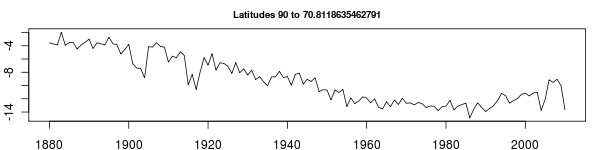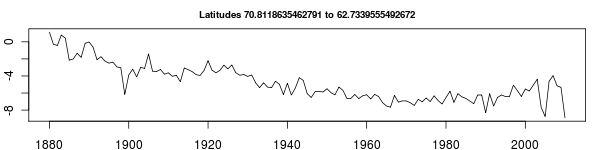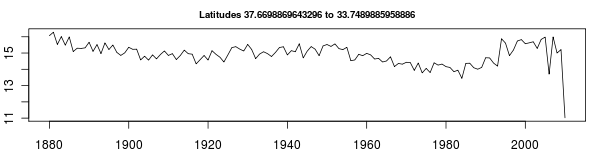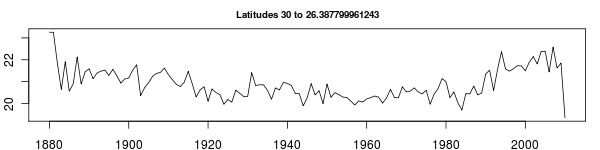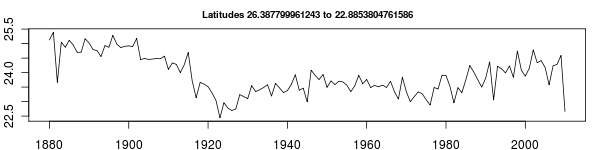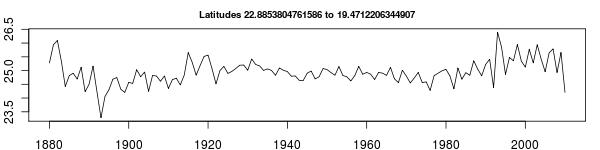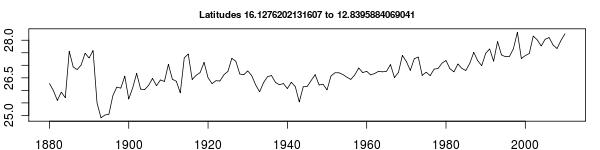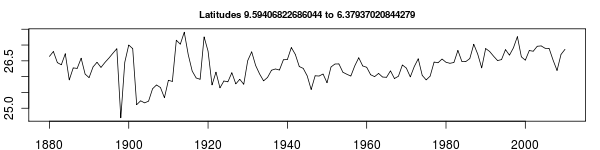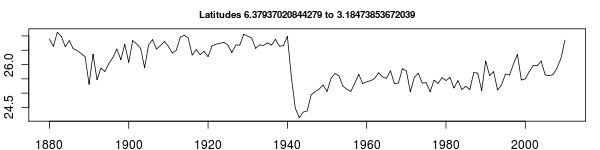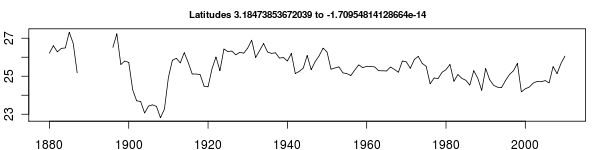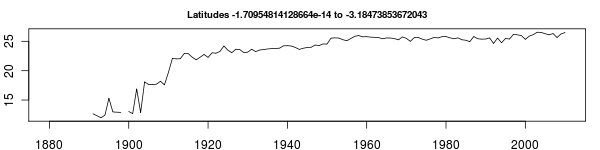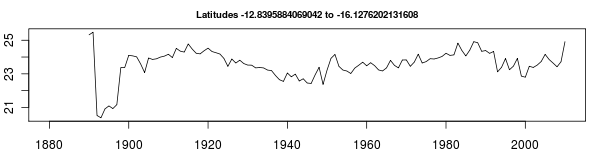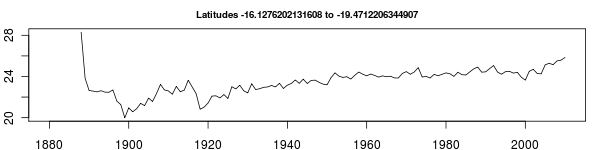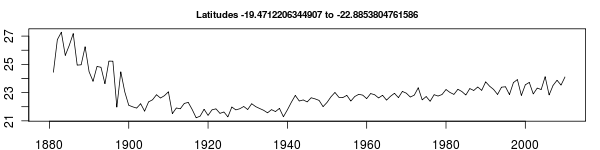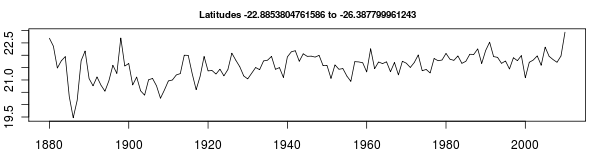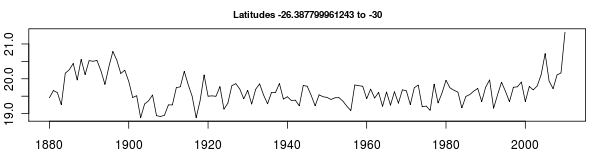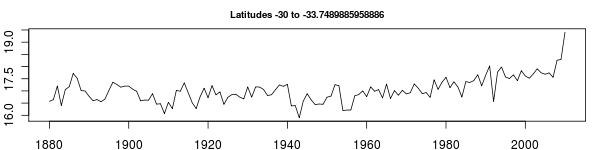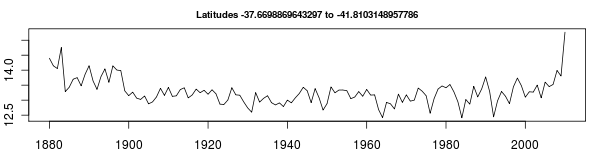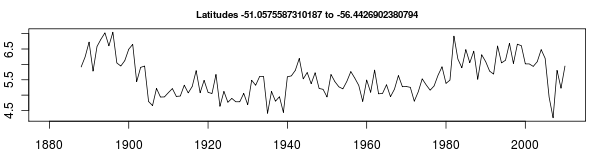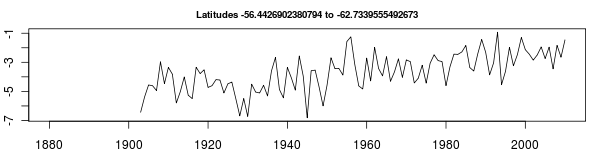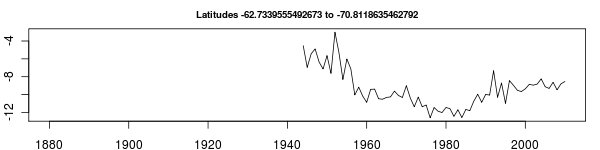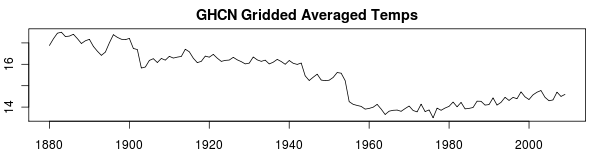1. 2010 July 18 at 6:54 pm

Not shabby!

My 5×5 grid model takes ~30 seconds to run in STATA, but thats only 648-odd gridcells.

2. 2010 July 19 at 7:05 am

5×5 => 648 grids?

5×5 should be 90N-90S = 36 zones
and by long 180W-180E = 72 zones
multiplied = 2592

648 is a 10×10 grid. Are you splitting the hemispheres first?

3. 2010 July 19 at 7:20 am

Nah, just failing at mental math :-p

4. 2010 July 19 at 8:39 am
5. 2010 July 19 at 11:29 am

Thanks Ron. Can you give me the key for the .inv file (e.g. what values correspond to what character range)? I’ve forgotten where/what everything is.

Something like:
country 1-3 station_id 4-11 state 13-14 name 15-42 lat 43-49 lon 50-57…

6. 2010 July 19 at 11:46 am

https://rhinohide.wordpress.com/ghcn-station-map/

With the modification that the state is prepended to the the station name
Also, the native USHCN id has been modified as follows:

```    USHCN:    011084-07
fake GHCN: 42501108407```

So those last digits are not ‘multiple records for same site’ or ‘dupe record.’

Just noticed that the alts are parsed wrong.
What’s more, this is all from the V1 station inventory.
Can’t update it to V2 until tomorrow

7. 2010 July 19 at 11:55 am

Yeh, I was noticing that some stations in the USHCN inventory file are not in your version.

station_id
[snip]

rb: yup – ushcn v1 not ushvn v2. Sorry about that. Update tomorrow.

8. 2010 July 19 at 9:56 pm

Same URLs, Zeke, but this time with USHCN v2 stations.

9. 2010 July 20 at 7:46 am

Thanks Ron. By the way, did you get the email from me and Matt about setting up a time for a call tomorrow or Thurs?

10. 2010 July 20 at 8:34 am

Yup – just confirmed that I’m free.

1. No trackbacks yet.